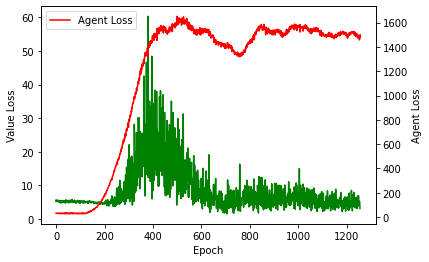# Policy iteration agent not improving

Hey everyone,

I am trying to implement an approach similar to this one: https://arxiv.org/abs/1912.01603

In the policy generation part, the agent tries to generate a policy by the returns of the environment model and the estimated values of a value network, which in turn computes the value of states based on the policy. However, my agent does not seem to be improving and its loss increases steadily because the value network assigns lower and lower values to the states. It seems like the agent converges to always output an action of 1.

Here is the important part of my code:

``````def agent_training(epochs):

for p in gen.parameters():

for epoch in range(epochs):

sample= data_agent.__next__().to(device)

try:

#check that it is the correct shape

sample = sample.view(agent_batches,d_state+d_action+d_state+3)

except:

continue

sample = sample[:,:d_state+1]

states = compute_transitions(sample)

value_estimates = compute_value_estimates(states)

train_action_model(value_estimates)

states = states[:,:,:d_state+1]

train_value_model(states.detach(),value_estimates.detach())

#plot losses

if epoch%20 == 0:

print(value_estimates[0,0],values(states)[0,0])

fig, ax1 = plt.subplots()

ax2 = ax1.twinx()

ax1.plot(range(len(v_losses)), v_losses, 'g', label = 'Value Loss')

ax2.plot(range(len(a_losses)), a_losses,'r', label="Agent Loss")

ax1.set_xlabel("Epoch")

ax1.set_ylabel("Value Loss")

ax2.set_ylabel("Agent Loss")

plt.legend()

plt.show()

del states

del value_estimates

def compute_transitions(states):

state_tensor = torch.empty(agent_batches,t,d_state+2).to(device)

s = states

for i in range(t):

n_batch = noise(agent_batches).to(device)

action = agent(s)

g_out = gen(n_batch,s,action)

s = g_out[:,:d_state+1].to(device)

state_tensor[:,i,:] = g_out[:,:d_state+2]

return state_tensor`

`def compute_value_estimates(states):

value_tensor = torch.empty(agent_batches,t,1).to(device)

for i in range(t):

value_tensor[:,i,:] = value_estimates(states,i)

return value_tensor`

def train_action_model(value_estimates):

#compute loss

loss = torch.mean(-torch.sum(value_estimates.squeeze(),dim=1))

a_losses.append(loss)

loss.backward()

a_optimizer.step()     `

`def train_value_model(states,value_estimates):

output = values(states)

loss_fn = torch.nn.MSELoss()

loss = loss_fn(value_estimates.squeeze(),output.squeeze())

v_losses.append(loss)

loss.backward()

v_optimizer.step()`

`def value_estimates(sample,steps_in_future):

v = torch.zeros(agent_batches,1).to(device)

for i in range(1,t):

v += ((1-lambda_v)*(lambda_v**(i-1))*value_estimates_k(sample,i,steps_in_future))

v += lambda_v**(t-1)*value_estimates_k(sample,t,t)

return v`

`def value_estimates_k(sample,k,steps_in_future):

v = torch.zeros(agent_batches,1).to(device)

h = min(k,t-steps_in_future)

for j in range(h):

reward = (sample[:,j,d_state+1]*(0.99**j))

v += reward.unsqueeze(dim=1)

estimate = values(sample[:,h,:d_state+1])

v = v+ (0.99**h)*estimate

return v

``````

I hope anyone can help my figure out why my code is not working.